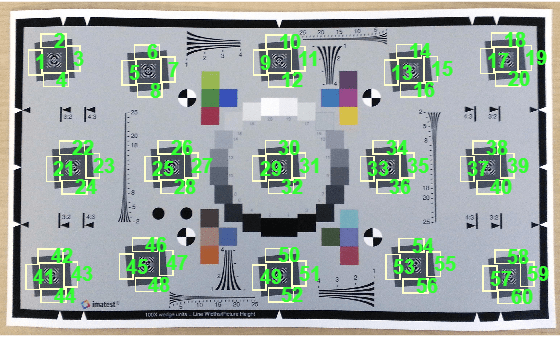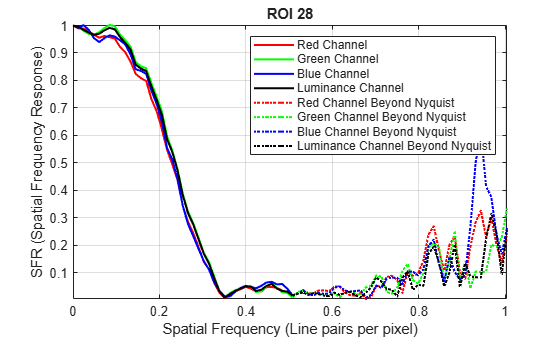Main Content

# measureSharpness

Measure spatial frequency response using Imatest eSFR chart

## Syntax

``sharpnessTable = measureSharpness(chart)``
``sharpnessTable = measureSharpness(chart,Name,Value)``
``[sharpnessTable,aggregateSharpnessTable] = measureSharpness(___)``

## Description

````sharpnessTable = measureSharpness(chart)` measures the spatial frequency response (SFR) at all slanted edge regions of interest (ROIs) of an Imatest® eSFR chart . The returned sharpness table includes the frequency for each ROI at which the response drops to 50% of the initial and peak values.```

example

````sharpnessTable = measureSharpness(chart,Name,Value)` measures the SFR at all specified slanted edge ROIs, specifying additional parameters.```
````[sharpnessTable,aggregateSharpnessTable] = measureSharpness(___)` also returns the average SFR of vertical and horizontal ROIs, using the input arguments of either of the previous syntaxes.```

## Examples

collapse all

Read an image of an eSFR chart into the workspace.

`I = imread('eSFRTestImage.jpg');`

Create an `esfrChart` object, then display the chart with ROI annotations. The 60 slanted edge ROIs are labeled with green numbers.

```chart = esfrChart(I); displayChart(chart,'displayColorROIs',false,... 'displayGrayROIs',false,'displayRegistrationPoints',false)```Measure the edge sharpness in ROIs 25-28, and return the measurements in `sharpnessTable`. Include measurements of the MTF70 and MTF30 by specifying the `'percentResponse'` name-value pair argument.

`sharpnessTable = measureSharpness(chart,'ROIIndex',25:28,'PercentResponse',[70 30])`
```sharpnessTable=4×9 table ROI slopeAngle confidenceFlag SFR comment MTF70 MTF70P MTF30 MTF30P ___ __________ ______________ ____________ ____________ ____________________________________________ ____________________________________________ ________________________________________ ________________________________________ 25 4.2268 true {85x5 table} {0x0 double} 0.061637 0.059828 0.053096 0.059827 0.061637 0.059828 0.053096 0.059827 0.107 0.1118 0.11037 0.11082 0.107 0.1118 0.11037 0.11082 26 5.0814 true {85x5 table} {0x0 double} 0.18553 0.18604 0.18528 0.18562 0.18553 0.18604 0.18528 0.18562 0.26282 0.26497 0.26211 0.26385 0.26282 0.26497 0.26211 0.26385 27 4.7787 true {85x5 table} {0x0 double} 0.069499 0.06935 0.063808 0.06899 0.069499 0.06935 0.063808 0.06899 0.21579 0.21794 0.21785 0.21775 0.21579 0.21794 0.21785 0.21775 28 4.7966 true {85x5 table} {0x0 double} 0.19057 0.20361 0.19589 0.20006 0.19057 0.20341 0.19565 0.20006 0.26185 0.27258 0.26126 0.26972 0.26185 0.27241 0.26118 0.26972 ```

Select the fourth row in the sharpness table, which corresponds to ROI 28. Display the SFR plot of the ROI.

```idx = 4; plotSFR(sharpnessTable(idx,:))```Print the MTF70 and MTF30 measurements of the ROI. Compare the measurements against the plot.

The MTF70 measurement of the red and blue color channels are slightly smaller than 0.2, while the MTF70 measurement of the green and luminance channels are slightly larger than 0.2. These measurements agree with a visual inspection of the SFR plot, on which an SFR value of 0.7 occurs at spatial frequencies around 0.2 line pairs per pixel.

`mtf70 = sharpnessTable.MTF70(idx,:)`
```mtf70 = 1×4 0.1906 0.2036 0.1959 0.2001 ```

The MTF30 measurement of the blue color channel is noticeably smaller than the MTF30 measurement of the other color channels. This measurement agrees with a visual inspection of the SFR plot, on which the SFR curve of the blue channel drops off more quickly than the other channels.

`mtf30 = sharpnessTable.MTF30(idx,:)`
```mtf30 = 1×4 0.2619 0.2726 0.2613 0.2697 ```

## Input Arguments

collapse all

eSFR chart, specified as an `esfrChart` object.

### Name-Value Pair Arguments

Specify optional comma-separated pairs of `Name,Value` arguments. `Name` is the argument name and `Value` is the corresponding value. `Name` must appear inside quotes. You can specify several name and value pair arguments in any order as `Name1,Value1,...,NameN,ValueN`.

Example: `'ROIIndex',2` measures the sharpness only of ROI 2.

ROI indices to include in measurements, specified as the comma-separated pair consisting of `'ROIIndex'` and a scalar or vector of integers in the range [1, 60]. The indices match the ROI numbers displayed by `displayChart`.

Note

`measureSharpness` uses the intersection of ROIs specified by `'ROIIndex'` and `'``ROIOrientation``'`.

Example: `29:32`

Data Types: `single` | `double` | `int8` | `int16` | `int32` | `uint8` | `uint16` | `uint32`

ROI orientation, specified as the comma-separated pair consisting of `'ROIOrientation'` and `'both'`, `'vertical'`, or `'horizontal'`. The `measureSharpness` function performs measurements only on ROIs with the specified orientation.

Note

`measureSharpness` uses the intersection of ROIs specified by `'``ROIIndex``'` and `'ROIOrientation'`.

Example: `'vertical'`

Data Types: `char` | `string`

Value of frequency response at which to report the corresponding spatial frequency, specified as the comma-separated pair consisting of `'PercentResponse'` and a scalar or vector of integers in the range [1, 100].

Each value of `PercentResponse` adds two columns to the `sharpnessTable` and `aggregateSharpnessTable` output arguments. The columns indicate the frequency at which the SFR drops to the specified percent of the initial and peak values. For example, when `PercentResponse` has the value 50, both output tables have the columns `MTF50` and `MTF50P`. These columns indicate the frequency at which the SFR drops to 50% of the initial value and peak value, respectively.

Example: `30`

Data Types: `single` | `double` | `int8` | `int16` | `int32` | `int64` | `uint8` | `uint16` | `uint32` | `uint64` | `logical` | `char` | `string`

## Output Arguments

collapse all

SFR measurements of edges, returned as an m-by-n table. m is the number of sampled ROIs. n changes values depending on `PercentResponse`. The first five columns are always present and represent these variables:

VariableDescription
`ROI`Index of the sampled ROI. The value of `ROI` is an integer in the range [1, 60].
`slopeAngle`Angle between the slanted edge and pure vertical or horizontal, depending on the ROI orientation. The angle is measured in degrees, and it is returned as a scalar of type `double`.
`confidenceFlag`

Boolean flag that indicates whether the sharpness measurement is reliable. `confidenceFlag` is `true` when the measurement is reliable. `confidenceFlag` is `false` when the measurement is unreliable due to the following conditions:

• `slopeAngle` is less than 3.5 degrees or more than 15 degrees.

• The contrast within the ROI is less than 20%.

The contrast of a slanted edge ROI is defined as ```100 * (IHigh - ILow)/(IHigh + ILow)```, where `IHigh` and `ILow` are the estimated average intensities of the high and low intensity regions across the edge. The contrast is computed for only the red channel.

`SFR`

Spatial frequency response of the edge in the ROI. `SFR` is an f-by-5 table. The five columns represent the frequency value and the red, green, blue, and luminance values corresponding to that frequency. f is the number of frequency samples of the MTF.

Luminance (Y) is a linear combination of the red (R), green (G), and blue (B) channels according to:

Y = 0.213R + 0.715G + 0.072B

`comment`When `confidenceFlag` is `false`, then `comment` describes the reason the measurement is unreliable. When `confidenceFlag` is `true`, then `comment` is the empty vector, `[]`.

Each value of `PercentResponse` adds two columns that indicate the frequency at which the SFR drops to the specified percent of the initial and peak value. The format of each entry in the column is a 1-by-4 vector. The four elements correspond to the red, green, blue, and luminance channels, respectively.

Average SFR measurements of vertical and horizontal edges, returned as a table with one or two rows. `aggregateSharpnessTable` has one row when all sampled ROIs have the same orientation. It has two rows when the sampled ROIs have mixed orientation. `aggregateSharpnessTable` has three fewer columns than `sharpnessTable`.

The first two columns of `aggregateSharpnessTable` are always present and represent these variables:

VariableDescription
`Orientation`Orientation of the averaged SFRs. The value of `Orientation` is either `'horizontal'` or `'vertical'`.
`SFR`

Averaged spatial frequency response of all edges in included ROIs with the orientation specified by `Orientation`.

`SFR` is an s-by-5 table. The five columns represent the frequency value, and the averaged red, green, blue, and luminance values corresponding to that frequency. s is the number of frequency samples of the MTF.

Luminance (Y) is computed as a linear combination of the red (R), green (G), and blue (B) channels according to:

Y = 0.213R + 0.715G + 0.072B

Each value of `PercentResponse` adds two columns that indicate the frequency at which the SFR drops to the specified percent of the initial and peak value. The format of each entry in the column is a 1-by-4 vector. The four elements correspond to the red, green, blue, and luminance channels, averaged among all sampled ROIs with the same orientation.

## Tips

• Slanted edges on a properly oriented chart are at an angle of 5 degrees from the horizontal or vertical. Sharpness measurements are not accurate when the edge orientation deviates significantly from 5 degrees.

• Sharpness is higher toward the center of the imaged region and decreases toward the periphery. Horizontal sharpness is usually higher than vertical sharpness.

## Algorithms

The SFR measurement algorithm is based on work by Peter Burns  . First, `measureSharpness` determines the edge position with sub-pixel resolution for each scan line, or row or column of pixels perpendicular to the edge, in the ROI. For example, each row of pixels is a scan line for a near-vertical edge. Next, `measureSharpness` aligns and averages the scan lines to create an oversampled edge intensity profile. The function takes the derivative of the intensity profile and applies a windowing function. The returned SFR measurement is the absolute value of the Fourier transform of the windowed derivative.

 Burns, Peter. "Slanted-Edge MTF for Digital Camera and Scanner Analysis." Society for Imaging Science and Technology; Proceedings of the Image Processing, Image Quality, Image Capture Systems Conference. Portland, Oregon, March 2000, pp. 135–138.

 Burns, Peter. "sfrmat3: SFR evaluation for digital cameras and scanners." URL: http://losburns.com/imaging/software/SFRedge/sfrmat3_post/index.html.

Download ebook